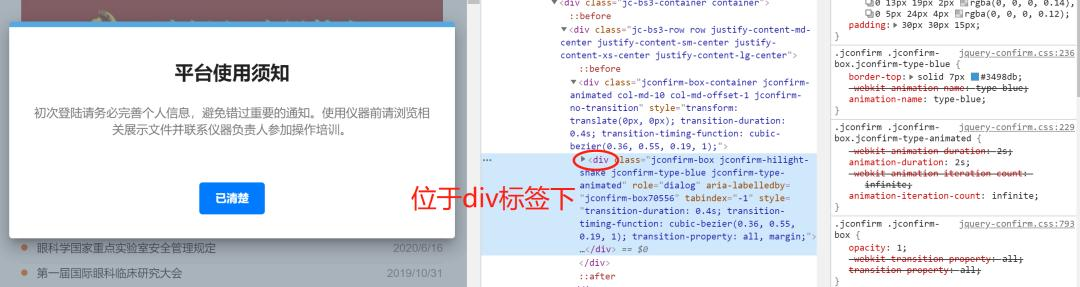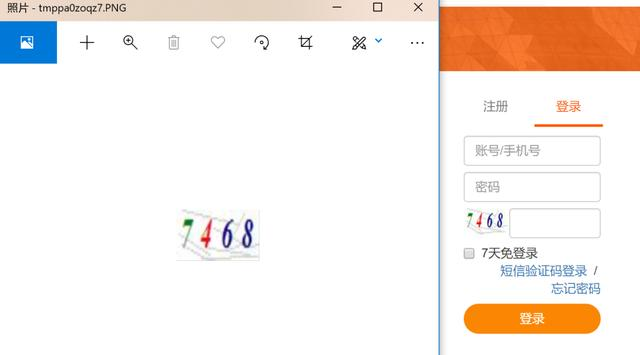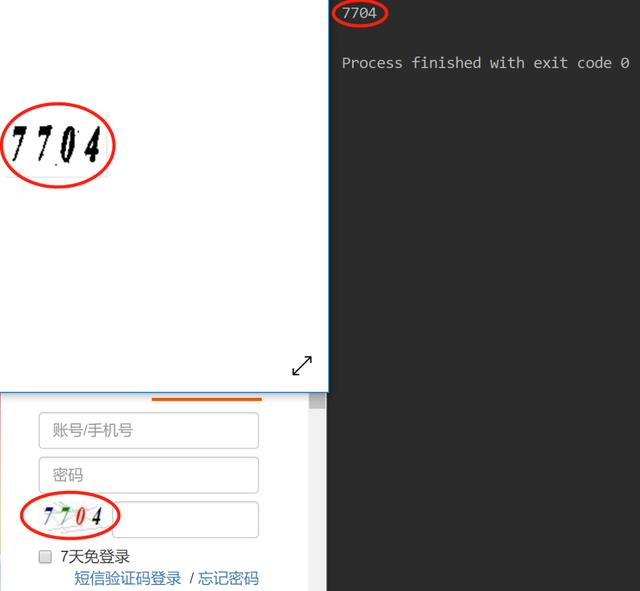##全国校区# 爬虫遇到头疼的验证码？python实战讲解弹窗处理和验证码识别2020-07-29

# 前言：selenium 和 tesseract 的配置读者可自行搜索，本文不做介绍)

# python实战

```import re
# 图片处理
from PIL import Image
# 文字识别
import pytesseract
# 浏览器自动化
from selenium import webdriver
import time```

# 解决弹出框问题

```url = 'http://lims.gzzoc.com/client'
driver = webdriver.Chrome()
driver.get(url)
time.sleep(30)```confirm(message)方法用于显示一个带有指定消息和 OK 及取消按钮的对话框

prompt(text,defaultText)方法用于显示可提示用户进行输入的对话框```url = 'http://lims.gzzoc.com/client'

driver = webdriver.Chrome()
driver.get(url)
time.sleep(1)
driver.maximize_window() # 最大化窗口
driver.find_element_by_xpath("//div[@class='jconfirm-buttons']/button").click()```

# 获取图片位置并截图

```img = driver.find_element_by_xpath('//img[@id="valiCode"]')
time.sleep(1)
location = img.location
size = img.size
# left = location['x']
# top = location['y']
# right = left + size['width']
# bottom = top + size['height']
left = 2 * location['x']
top = 2 * location['y']
right = left + 2 * size['width'] - 10
bottom = top + 2 * size['height'] - 10
driver.save_screenshot('valicode.png')
page_snap_obj = Image.open('valicode.png')
image_obj = page_snap_obj.crop((left, top, right, bottom))
image_obj.show()```# 验证码图片的进一步处理

```img = image_obj.convert("L")  # 转灰度图
w, h = img.size
threshold = 205
# 遍历所有像素，大于阈值的为黑色
for y in range(h):
for x in range(w):
if pixdata[x, y] < threshold:
pixdata[x, y] = 0
else:
pixdata[x, y] = 255```

```data = img.getdata()
w, h = img.size
black_point = 0
for x in range(1, w - 1):
for y in range(1, h - 1):
mid_pixel = data[w * y + x]
if mid_pixel < 50:
top_pixel = data[w * (y - 1) + x]
left_pixel = data[w * y + (x - 1)]
down_pixel = data[w * (y + 1) + x]
right_pixel = data[w * y + (x + 1)]
if top_pixel < 10:
black_point += 1
if left_pixel < 10:
black_point += 1
if down_pixel < 10:
black_point += 1
if right_pixel < 10:
black_point += 1
if black_point < 1:
img.putpixel((x, y), 255)
black_point = 0
img.show()```# 文字识别

```result = pytesseract.image_to_string(img)
# 可能存在异常符号，用正则提取其中的数字
regex = '\d+'
result = ''.join(re.findall(regex, result))
print(result)```# 提交账号密码、验证码等信息

```driver.find_element_by_name('code').send_keys(result)
# 最后点击确定

```while True:
try:
...
break
except:
driver.find_element_by_id('valiCode').click()```

# 小结

OK